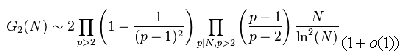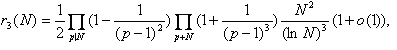• 汇集公众科学智慧交流科学思想见解
• 点燃科学智慧火花构建互动交流平台## 解决“哥得巴赫猜想”的必由之路 ——着重研究“哥得巴赫函数”

“哥得巴赫猜想”的原意是“对于充分大的偶数，至少可以表为一对素数之和”。

（A）每一个不小于6的偶数都是两个奇素数之和。

B）每一个不小于9的奇数都是三个奇素数之和。

C）存在一个正整数c,使每一个不小于2的整数都可以表示为不超过c个素数之和。(1912年，朗道)

DDhardy，当2|N.1922年，哈代与利特伍德猜想

E2N

SELBERG(N):Dselberg(n)<=16*C1(N)*N/log(N)^2*(1+loglog(N)/log(N))（赛尔贝格公式）

WAN(N):Dwan(N)<=8*C1(N)*N/log(N)^2*(1+loglog(N)/log(N))（王元公式）

PAN(N):Dpan(N)<=12*C1(N)*N/log(N)^2*(1+loglog(N)/log(N))（潘承洞公式）

CHEN(N):Dchen(N)<=7.8342*C1(N)*N/log(N)^2（陈景润公式）

VUANHAN:Dvuanhan(N)<=SQR(N)（沃因公式）

HUA(N):Dhua(N)~HARDY(N)+O1(N)（华罗庚公式）